NEET  >  Case Based Questions Test: Units & Measurement

# Case Based Questions Test: Units & Measurement - NEET

Test Description

## 10 Questions MCQ Test Physics Class 11 - Case Based Questions Test: Units & Measurement

Case Based Questions Test: Units & Measurement for NEET 2023 is part of Physics Class 11 preparation. The Case Based Questions Test: Units & Measurement questions and answers have been prepared according to the NEET exam syllabus.The Case Based Questions Test: Units & Measurement MCQs are made for NEET 2023 Exam. Find important definitions, questions, notes, meanings, examples, exercises, MCQs and online tests for Case Based Questions Test: Units & Measurement below.
Solutions of Case Based Questions Test: Units & Measurement questions in English are available as part of our Physics Class 11 for NEET & Case Based Questions Test: Units & Measurement solutions in Hindi for Physics Class 11 course. Download more important topics, notes, lectures and mock test series for NEET Exam by signing up for free. Attempt Case Based Questions Test: Units & Measurement | 10 questions in 20 minutes | Mock test for NEET preparation | Free important questions MCQ to study Physics Class 11 for NEET Exam | Download free PDF with solutions
 1 Crore+ students have signed up on EduRev. Have you?
Case Based Questions Test: Units & Measurement - Question 1

### Measurement of the time period of a simple pendulum and the associated errors:5 students were asked to measure the time period of a pendulum. They obtained the following result: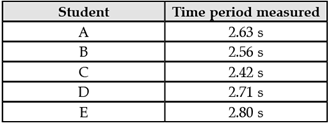The teacher explained 4 different types of errors involved in their observations:Absolute error: It is the difference of the arithmetic value of all the measured values and each of the measured values.Mean absolute error: It is the arithmetic mean of the value of absolute errors.Fractional error: It is the ratio of the mean absolute error to the arithmetic mean of the measured values.Percentage error: It is the fractional error multiplied by 100.What is the mean time period of oscillation of the pendulum?

Detailed Solution for Case Based Questions Test: Units & Measurement - Question 1
Mean time period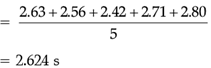Case Based Questions Test: Units & Measurement - Question 2

### Measurement of the time period of a simple pendulum and the associated errors:5 students were asked to measure the time period of a pendulum. They obtained the following result:The teacher explained 4 different types of errors involved in their observations:Absolute error: It is the difference of the arithmetic value of all the measured values and each of the measured values.Mean absolute error: It is the arithmetic mean of the value of absolute errors.Fractional error: It is the ratio of the mean absolute error to the arithmetic mean of the measured values.Percentage error: It is the fractional error multiplied by 100.What is the value of the mean absolute error?

Detailed Solution for Case Based Questions Test: Units & Measurement - Question 2
Mean absolute error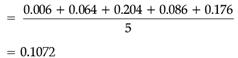Case Based Questions Test: Units & Measurement - Question 3

### Measurement of the time period of a simple pendulum and the associated errors:5 students were asked to measure the time period of a pendulum. They obtained the following result:The teacher explained 4 different types of errors involved in their observations:Absolute error: It is the difference of the arithmetic value of all the measured values and each of the measured values.Mean absolute error: It is the arithmetic mean of the value of absolute errors.Fractional error: It is the ratio of the mean absolute error to the arithmetic mean of the measured values.Percentage error: It is the fractional error multiplied by 100.The time period of the pendulum may lie between

Detailed Solution for Case Based Questions Test: Units & Measurement - Question 3
The mean absolute error is known as limit of error. If X is the mean measured value of a parameter and ΔX is the mean absolute error, then the magnitude of the parameter will lie between X + ΔX and X – ΔX.
Case Based Questions Test: Units & Measurement - Question 4

Vernier calipers:

Vernier calipers are used to measure the thickness of thin plates, diameters of small cylinders etc. It has two scales–one main scale and a Vernier scale, which slides along the main scale. The main scale and Vernier scale are divided into small divisions though of different magnitudes. The difference in the magnitude of one main scale division (M.S.D.) and one vernier scale division (V.S.D.) is called the least count of the instrument, as it is the smallest distance that can be measured using the instrument. It is also known as vernier constant. Least count may also be defined as: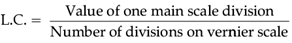When the jaws of vernier calipers touch each other, the zero of the Vernier should coincide with the zero of the main scale. If it is not so, then the instrument is said to have zero error. Zero error may be positive or negative, depending upon whether the zero of the vernier scale lies to the right or to the left of the zero of the main scale.

The least count of the instrument when 1MSD = 0.1 cm and number of vernier scale division = 10 is

Detailed Solution for Case Based Questions Test: Units & Measurement - Question 4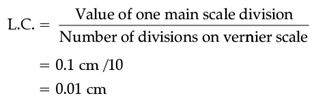Case Based Questions Test: Units & Measurement - Question 5

Vernier calipers:

Vernier calipers are used to measure the thickness of thin plates, diameters of small cylinders etc. It has two scales–one main scale and a Vernier scale, which slides along the main scale. The main scale and Vernier scale are divided into small divisions though of different magnitudes. The difference in the magnitude of one main scale division (M.S.D.) and one vernier scale division (V.S.D.) is called the least count of the instrument, as it is the smallest distance that can be measured using the instrument. It is also known as vernier constant. Least count may also be defined as:When the jaws of vernier calipers touch each other, the zero of the Vernier should coincide with the zero of the main scale. If it is not so, then the instrument is said to have zero error. Zero error may be positive or negative, depending upon whether the zero of the vernier scale lies to the right or to the left of the zero of the main scale.

Vernier constant is defined as

Detailed Solution for Case Based Questions Test: Units & Measurement - Question 5
Least count of Vernier Callipers is also known as Vernier Constant.

L.C. = V.C. = 1 M.S.D. – 1 V.S.D.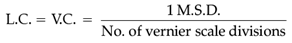Case Based Questions Test: Units & Measurement - Question 6

Vernier calipers:

Vernier calipers are used to measure the thickness of thin plates, diameters of small cylinders etc. It has two scales–one main scale and a Vernier scale, which slides along the main scale. The main scale and Vernier scale are divided into small divisions though of different magnitudes. The difference in the magnitude of one main scale division (M.S.D.) and one vernier scale division (V.S.D.) is called the least count of the instrument, as it is the smallest distance that can be measured using the instrument. It is also known as vernier constant. Least count may also be defined as:When the jaws of vernier calipers touch each other, the zero of the Vernier should coincide with the zero of the main scale. If it is not so, then the instrument is said to have zero error. Zero error may be positive or negative, depending upon whether the zero of the vernier scale lies to the right or to the left of the zero of the main scale.

Which of the following figures has no zero error?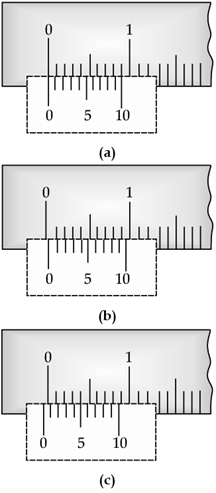Detailed Solution for Case Based Questions Test: Units & Measurement - Question 6
When the jaws of vernier calipers touch each other, the zero of the vernier should coincide with the zero of the main scale. In that case it is said to have no zero error.
Case Based Questions Test: Units & Measurement - Question 7

Vernier calipers:

Vernier calipers are used to measure the thickness of thin plates, diameters of small cylinders etc. It has two scales–one main scale and a Vernier scale, which slides along the main scale. The main scale and Vernier scale are divided into small divisions though of different magnitudes. The difference in the magnitude of one main scale division (M.S.D.) and one vernier scale division (V.S.D.) is called the least count of the instrument, as it is the smallest distance that can be measured using the instrument. It is also known as vernier constant. Least count may also be defined as:When the jaws of vernier calipers touch each other, the zero of the Vernier should coincide with the zero of the main scale. If it is not so, then the instrument is said to have zero error. Zero error may be positive or negative, depending upon whether the zero of the vernier scale lies to the right or to the left of the zero of the main scale.

Which figure shows positive zero error and which one shows positive zero error?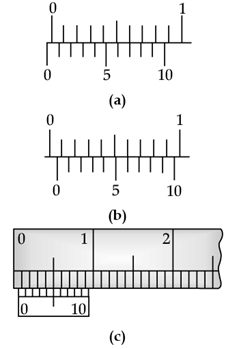Detailed Solution for Case Based Questions Test: Units & Measurement - Question 7
Zero error is positive when the zero of vernier scale lies to the right of the zero of the main scale.
Case Based Questions Test: Units & Measurement - Question 8

Vernier calipers:

Vernier calipers are used to measure the thickness of thin plates, diameters of small cylinders etc. It has two scales–one main scale and a Vernier scale, which slides along the main scale. The main scale and Vernier scale are divided into small divisions though of different magnitudes. The difference in the magnitude of one main scale division (M.S.D.) and one vernier scale division (V.S.D.) is called the least count of the instrument, as it is the smallest distance that can be measured using the instrument. It is also known as vernier constant. Least count may also be defined as:When the jaws of vernier calipers touch each other, the zero of the Vernier should coincide with the zero of the main scale. If it is not so, then the instrument is said to have zero error. Zero error may be positive or negative, depending upon whether the zero of the vernier scale lies to the right or to the left of the zero of the main scale.

A vernier callipers has vernier divisions = 10 and least count = 0.2 mm. What is the smallest distance can measured by this instrument?

Detailed Solution for Case Based Questions Test: Units & Measurement - Question 8
Least count of an instrument is the smallest distance that can be measured using the instrument.
Case Based Questions Test: Units & Measurement - Question 9

Measurement of the time period of a simple pendulum and the associated errors:

5 students were asked to measure the time period of a pendulum. They obtained the following result:The teacher explained 4 different types of errors involved in their observations:

Absolute error: It is the difference of the arithmetic value of all the measured values and each of the measured values.

Mean absolute error: It is the arithmetic mean of the value of absolute errors.

Fractional error: It is the ratio of the mean absolute error to the arithmetic mean of the measured values.

Percentage error: It is the fractional error multiplied by 100.

What are the absolute errors of student A and B?

Detailed Solution for Case Based Questions Test: Units & Measurement - Question 9
Absolute error of student A

= 2.624 – 2.63 = – 0.006

Absolute error of student B

= 2.624 – 2.56 = 0.064

Case Based Questions Test: Units & Measurement - Question 10

Measurement of the time period of a simple pendulum and the associated errors:

5 students were asked to measure the time period of a pendulum. They obtained the following result:The teacher explained 4 different types of errors involved in their observations:

Absolute error: It is the difference of the arithmetic value of all the measured values and each of the measured values.

Mean absolute error: It is the arithmetic mean of the value of absolute errors.

Fractional error: It is the ratio of the mean absolute error to the arithmetic mean of the measured values.

Percentage error: It is the fractional error multiplied by 100.

What is the value of the fractional error?

Detailed Solution for Case Based Questions Test: Units & Measurement - Question 10
Fractional error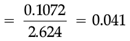## Physics Class 11

130 videos|483 docs|210 tests
Information about Case Based Questions Test: Units & Measurement Page
In this test you can find the Exam questions for Case Based Questions Test: Units & Measurement solved & explained in the simplest way possible. Besides giving Questions and answers for Case Based Questions Test: Units & Measurement, EduRev gives you an ample number of Online tests for practice

## Physics Class 11

130 videos|483 docs|210 tests

### How to Prepare for NEET

Read our guide to prepare for NEET which is created by Toppers & the best Teachers(Scan QR code)